# Slow saving in banks

How long will it take to save € 9,000 by depositing € 200 at the beginning of each year at 2% interest?

y =  32

### Step-by-step explanation: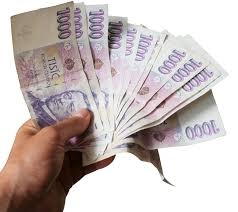Did you find an error or inaccuracy? Feel free to write us. Thank you!Tips to related online calculators
Do you want to convert time units like minutes to seconds?

## Related math problems and questions:

• DepositIf you deposit 719 euros at the beginning of each year, how much money we have at 1.3% (compound) interest after 9 years?
• SavingsThe depositor regularly wants to invest the same amount of money in the financial institution at the beginning of the year and wants to save 10,000 euros at the end of the tenth year. What amount should he deposit if the annual interest rate for the annua
• Mortage hypo loan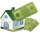The Jonáš family decided to buy an older apartment, which cost EUR 30,000. They found EUR 17,000 and took the loan with the bank for the remaining amount. What interest did they receive if they repay this amount for 15 years at EUR 120 per month?
• Compound interest 4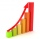3600 dollars is placed in an account with an annual interest rate of 9%. How much will be in the account after 25 years, to the nearest cent?
• Compound interest 3After 8 years, what is the total amount of a compound interest investment of $25,000 at 3% interest, compounded quarterly? (interest is now dream - in the year 2019) • Interst on savings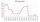The bank offers 1.6% interest. How many euros have to insert at the beginning if we received € 15 on the interest? • The depositDeposit has been placed for one year at an annual rate of 4.5%. After the added interst amount rose to € 2,612.5. Determine initial deposit. • Compound interestCompound interest: Clara deposited CZK 100,000 in the bank with an annual interest rate of 1.5%. Both money and interest remain deposited in the bank. How many CZK will be in the bank after 3 years? • Deposit 3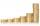After a year, Martin had on bank account € 2028. What was the interest rate when the initial deposit was € 1879? • Semiannually compound interestIf you deposit$5000 into an account paying 8.25% annual interest compounded semiannually, how long until there is $9350 in the account? • Millionaire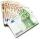Mr. Smith have gone withdraw from bank saving interest € 1,500. How big was his initial deposit if the annual interest rate is 1.5%? • Two accounts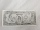Two accounts in the bank, one per year interest 2%, the second 3%. Total interest income 1900 USD. If interest rates were reversed, the yield would be USD 200 higher. What are the amounts on each account? • If you 3If you deposit$4500 at 5% annual interest compound quarterly, how much money will be in the account after 10 years?
• Investment1000\$ is invested at 10% compound interest. What factor is the capital multiplied by each year? How much will be there after n=12 years?
• Repay, interest, loanRamchacha takes a loan amount of 240000 from a bank for constructing a house at the rate of simple interest of 12% per annum. After 1 yr. of taking the loan he rents the house at the rate of 5200 per month. Determine the number of years he would take to r
• BankPaul put 10000 in the bank for 6 years. Calculate how much you will have in the bank if he not pick earned interest or change deposit conditions. The annual interest rate is 3.5%, and the tax on interest is 10%.
• Bank deposit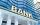Ms Jana after the withdraw amount of 2,500 euros after year saving in bank. What was her deposit if the interest rate was 2.5% p.a..